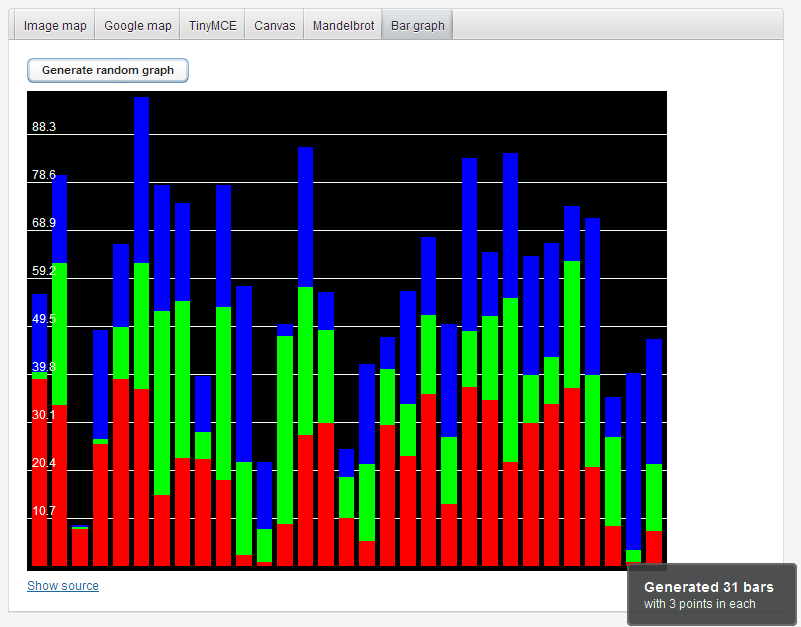Subscription# Directory

← Back

## BarGraph

An addon that draws (optionally stacked) bar graphs.

### RatingSupports automatic scaling, horizontal guidelines and a informative mouseover labels on the bars. The bars are drawn as plain divs.

## Sample code

```BarGraph graph = new BarGraph();
graph.setWidth("640px");
graph.setHeight("480px");

// Generate 20 random bars that have 2 datapoints each
List<Datapoint> data = new ArrayList<Datapoint>();
for (int i = 0; i < 20; ++i) {
Double[] points = new Double;
String[] labels = new String;
points = new Random().nextDouble() * 10;
labels = "Bar " + i + " point 1 value is " + points;
points = new Random().nextDouble() * 10;
labels = "Bar " + i + "  point 2 value is " + points;
data.add(new Datapoint("Bar " + i, points, labels));
}

graph.setData(data);```

### Version

• Fixed a Google App Engine compatability problem
• Fixed a problem with the empty constructor
Released
2010-05-02
Maturity
STABLE